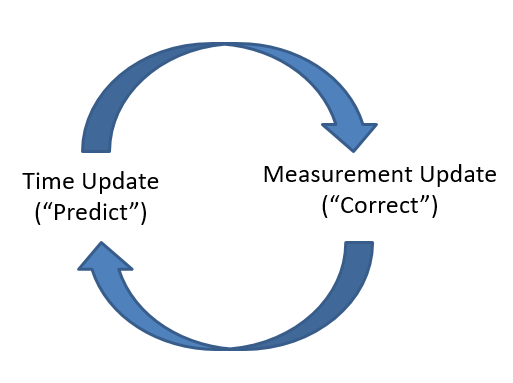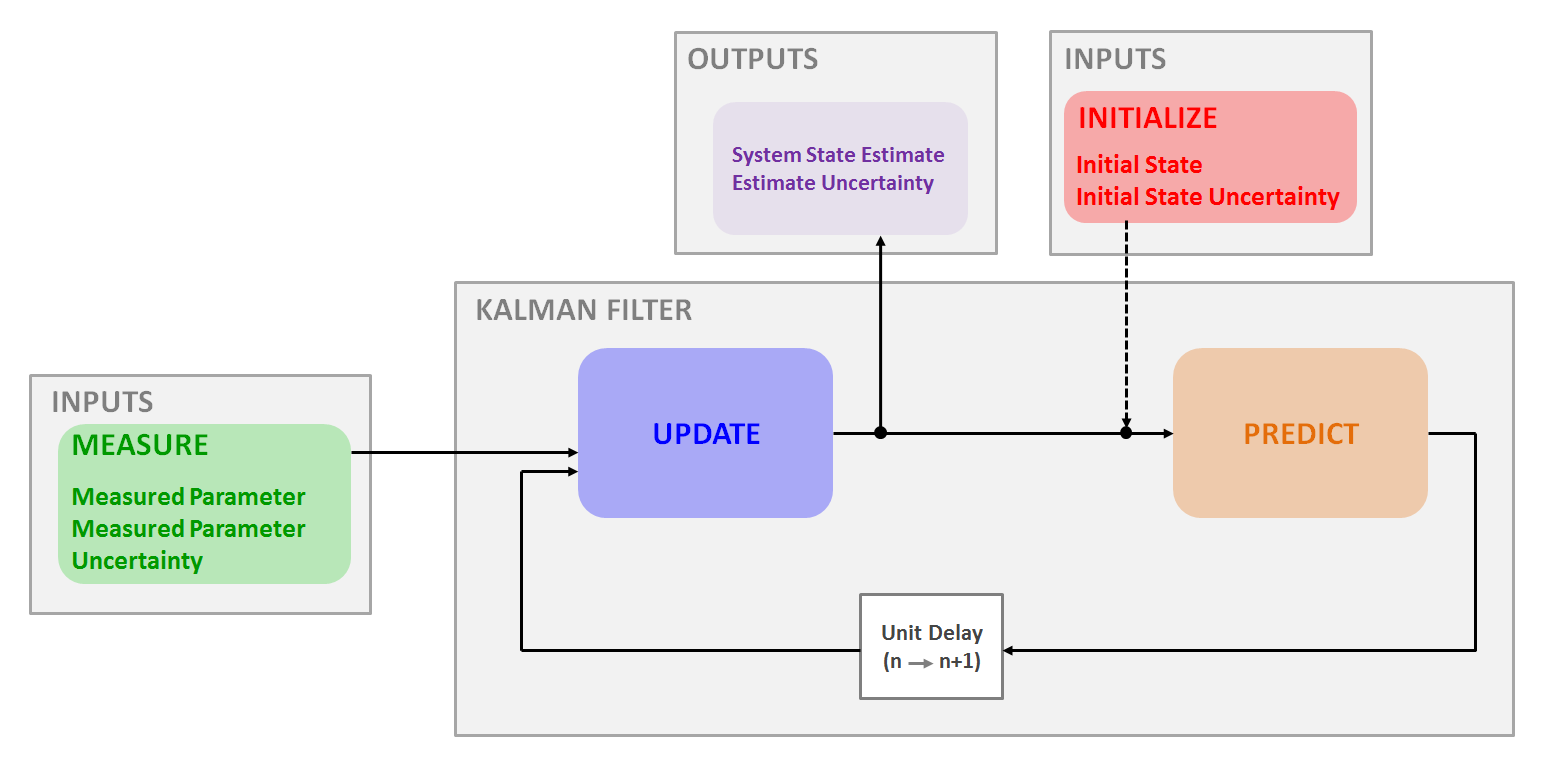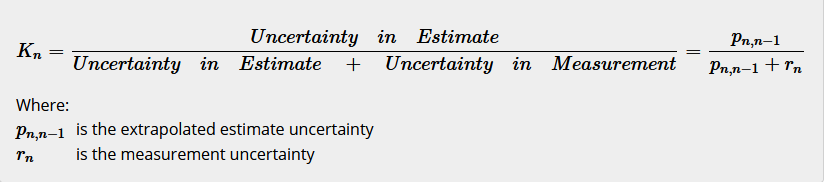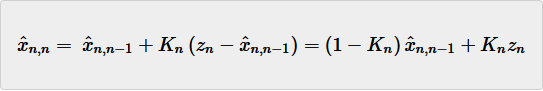# How I Wrote A Background Noise Remover from Start to Finish

## The story of my fall into the rabbit hole of linear algebra and how I got outVarun

Published on Mar 31, 2021

How do so many communication apps, like Signal and Telegram, get rid of all the static-y noise and background noise before the sound reaches the other people on the call?

These companies include noise removal in their software, and in a recent coding project, I wanted to do just that.

In this article, I will break down what I needed, how I made it, and the obstacles I faced along the way.

When I say ambient noise, background noise, or simply, noise, I am talking about sound that you do not want to hear in an audio file. This sound disrupts the signal, sound you do want to hear, in an audio file or incoming sound.

My plan was to somehow identify the noise so I could filter it out, then write the noise-removed-audio file to an "output" file once the program did its main functions so that I could compare and collect data.

# Research

Researching this was hard. It took me a while, but I found a tutorial on the Kalman Filter. This explained all the background knowledge needed to understand the Kalman Filter, explained the Kalman Filter, and gave examples.

The Kalman Filter has two types: One-Dimensional and Multi-Dimensional. The Multi-Dimensional Kalman Filter involves linear algebra concepts that I had to learn. So, I started learning linear algebra concepts and buried myself in lengthy research papers on the covariance matrix and eigenvectors.

I eventually found that the only concepts needed for the Kalman Filter with regards to linear algebra were matrices, vectors, and their operations. I also found a helpful video on the covariance matrix that explained what the research papers were describing, in 10 minutes.

In addition, I learned the Java Sound API on Oracle's official programming guide so that I could use Java to apply the Kalman Filter to sound. Unfortunately, the Java Sound API did not provide the low-level functions that I needed. The only useful function was the byte data, which is binary data that represents the sound, of the audio file. I then had to use Linear Pulse Code Modulation to determine the sound wave, and then apply the Kalman Filter from there.

## Kalman Filter

The Kalman Filter is an estimation algorithm that lets you find the value of an object that you may never know for sure, called a "hidden state" but get very close to it by measuring the object and going through a series of equations that lets you converge to the true value.

For example, if you want to use the Kalman Filter to find the weight of a bronze Trojan horse, its hidden state might be 200kg (because of all the men inside) and your final state estimate, which is what you get when you put your measurement through a bunch of equations, is 198.4 kg.

In the Kalman Filter, every time you measure, you update your state estimate using an equation. Then, you predict the next state estimate, which you calculate after your next measurement.The Kalman Filter uses the prediction-correction mechanism.The Kalman Filter follows the "measure, update, predict" cycle.

### How it Works

Going back to the Trojan Horse example, let's say you set your initial guess to 100kg. The sole purpose of the initial guess is to get the cycle started.

In addition, you need to set your initial guess for the estimate uncertainty. In other words, use a number between 0 and 1 representing how uncertain you are about 100kg being the hidden state.

Then, you finish the "0th" iteration of the Measure, Update, Predict cycle. These initial guesses are the "estimates," so now you need to make the prediction. Because you don't have a measurement yet, the predictions are the same as the estimate, and they are going to be used in the 1st iteration.

1. You put the Trojan Horse on a scale and you see "195kg." This is your measurement

2. Calculate the Kalman Gain. As this factor increases, the measurement is given more weight and as it decreases, the measurement is given less weight and the estimate is given more weight, as shown below.Kalman Gain Equation

The Kalman Gain uses the estimate uncertainty and the measurement uncertainty in its calculation. The measurement uncertainty is a constant value between 0 and 1 that you set in the beginning.

1. Now, plugging in the prediction from the 0th iteration (the previous state prediction) - 100kg - and your current measurement - 195kg - into the State Update Equation, you get your state estimate.State Update Equation

1. Next, you update the estimate uncertainty with another equation, the Covariance Update Equation.

2. Finally, you predict the next estimate and the next estimate uncertainty using the final 2 equations in the Kalman Filter algorithm.

And that's the cycle for the One Dimensional Kalman Filter. You can make it even simpler by ignoring the uncertainty. You now make another measurement and keep cycling through until you've made all the measurements you would like.

When you graph the estimate and hidden state as the y values and the iteration # as the x-value, you'll see the estimate converge to the true value.

### Implementing the Kalman Filter

The kalmanfilter package was the first module I worked on after experiment a little bit with the Java Sound API (and mostly failing). Because I love to code, I didn't even implement the Kalman Filter first. I implemented simpler filters that also follow the "measure, update, predict" cycle: `AlphaFilter`, `AlphaBetaFilter`, and `AlphaBetaGammaFilter`.

Following test-driven development, I tested these with the values in the examples from the same site that taught me the filters themselves: kalmanfilter.net. I made the right decision testing, for I immediately faced lots of failing tests because of lots of bugs.

In addition to giving me testing experience, of which I have very little, implementing similar and simpler filters helped me hammer out the bugs that would likely have appeared in the Kalman Filter had I started with that. Starting off simpler let me focus on the more complicated bugs when I finally got to the more complicated filters.

## Linear Pulse Code Modulation and Sound Manipulation

Sound files use a format called Linear Pulse Code Modulation to represent sound as numbers. At a specified sample rate, the bytes of data are specific amplitudes, and together they make sine waves, which is how sound is represented.

At first, I thought that the byte data itself were the samples that could be directly converted to sine waves. When the audio file was corrupted and produced unintelligible noise.

More than two months into my project, I discovered an algorithm on Stack Overflow that let me abstract the [bitwise operations], the intermediate step between the byte data and the sine waves which I knew how to manipulate.

And these worked like a charm! Increase the a value (amplitude) and its louder, increase the product inside the `Math.sin()` and the pitch goes higher. I could then combine `float` (a data type for decimal numbers) samples together to add sine or sound waves.

And when I added an amplitude and its negative, I got the expected silence. This is called destructive interference - when you add a sound sine wave and the inverse sine wave together. In mathematical terms, you got a horizontal line, and in sound terms, that's complete silence.

## Modeling Matrices and Vectors

The multidimensional Kalman Filter uses matrices), which are rectangular arrays of numbers, and vectors, which are single-dimensional arrays of numbers. I had the most fun building this because I was working with a concept that I had already been introduced to in school, and I was using Java syntax that I was familiar with.

I created a Matrix class that held a two dimensional array for the matrix and a Vector class that extends the Matrix class and held a one dimensional array.

Following test-driven development, I first made a Matrix test class and VectorTest class.

For the matrix addition test, I called my `Matrix#add()` method on the first matrix passing in the second matrix. I then checked if the sum matrix was the correct value by creating a third `Matrix` object.

``````@Test
Matrix addend = new Matrix(new double[][] { { 1, 2, 3 }, { 4, 5, 6 } });
Matrix augend = new Matrix(new double[][] { { 10, 14, -8 }, { 3, 1, 5 } });

assertArrayEquals(new double[][] { { 11, 16, -5 }, { 7, 6, 11 } }, sum.getMatrixElements());
}
``````
``````public Matrix plus(Matrix augend) {
if (!(getRows() == augend.getRows() && getColumns() == augend.getColumns()))
throw new IllegalArgumentException("Cannot add matrices of different dimensions");
Matrix toReturn = new Matrix(new double[getRows()][getColumns()]);

for (int i = 0; i < getRows(); i++) {
for (int j = 0; j < getColumns(); j++) {
toReturn.set(i, j, matrixElements[i][j] + augend.getMatrixElements()[i][j]);
}
}

}
``````

After implementing `Matrix#add`, I continued for the subtraction, multiplication, inverse (which was so complicated I ended up extracting into its own class), and transpose operations, each time testing before implementing. I also included various methods concerning properties of the matrix, such as

• `getDeterminant()`
• `isSquare()`
• `isIdentityMatrix()`
• `isInverse(Matrix inverse)`

Finally, I implemented static factory methods for generic matrix types, which right now is only for the identity matrix, which is where 1's span the major diagonal and everything else in the matrix is a zero, and it is a square matrix. For example, the 3x3 identity matrix is [[1,0,0],[0,1,0],[0,0,1]]

## Results

When I got rid of all `Math.random()`s, `NullPointerException`s and `UnsupportedOperationException`s, which stopped my program from fully running, I found no change in the audio file.

## Conclusion

Ambient Noise Remover was a huge challenge, and I have not successfully removed background noise, yet. I, however, learned so much from the experience, from sound engineering to linear algebra to Java APIs.

Here is the link to the code (open source!): github.com/Ambient-Noise-Remover

It is also available as a JAR file, just make sure you have installed the Java JDK before downloading my program!

If you'd like to learn the Kalman Filter in detail, I suggest the tutorial website (kalmanfilter.net), as it explains everything very simply.

Thanks for reading my article! Do you have a project that involves a complex math topic, such as linear algebra? Leave a link to it in the comments!